LEARNING MATHS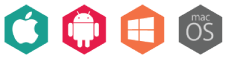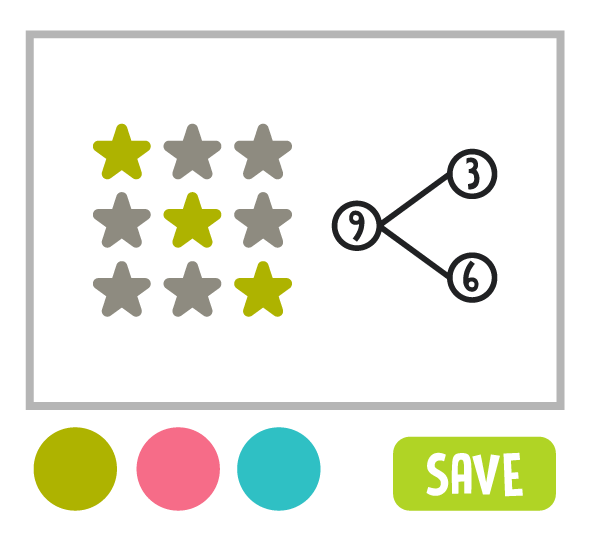SAP Learning Maths is a brilliant interactive workbook that makes math easy to understand for kids who are having learning difficulties in math.

It provides both teachers/parents/guardians and students a summary of learning objectives, formulae sheets for checking/recalling math formulae, work exercises for becoming familiar with various questions, non-routine questions for developing analytical skills and carefully worked out step-by-step solutions.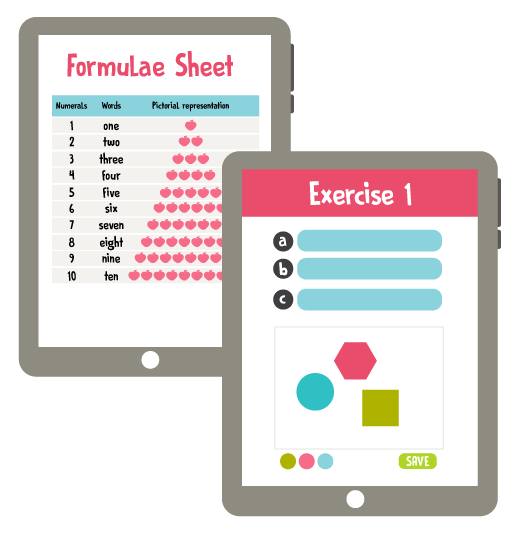What's amazing about this courseware is the gradual presentation of lessons and the numerous practice tests it included that will facilitate better absorption of the mathematical lessons for young minds. The questions posted will also ensure that multiple concepts are understood.

Give your child the gift of easy learning for their math subject with Learning Maths now!

CONTENT PREVIEW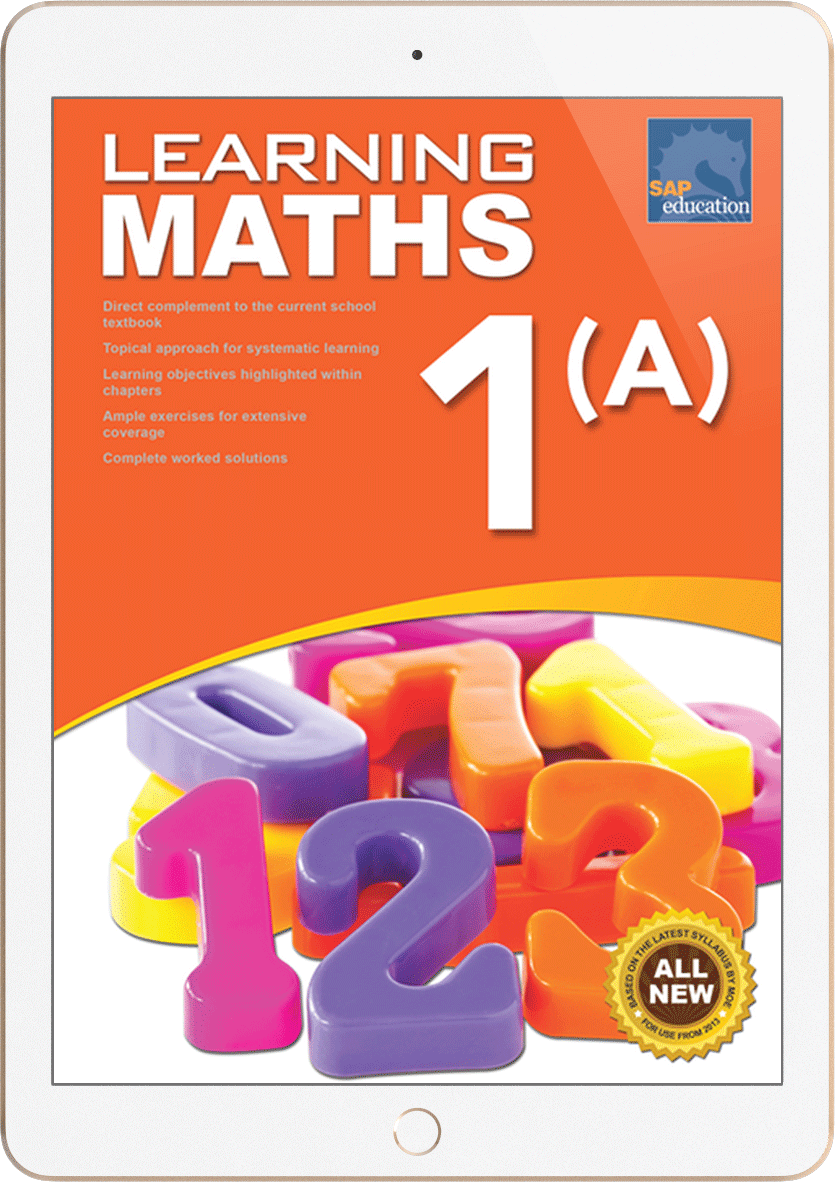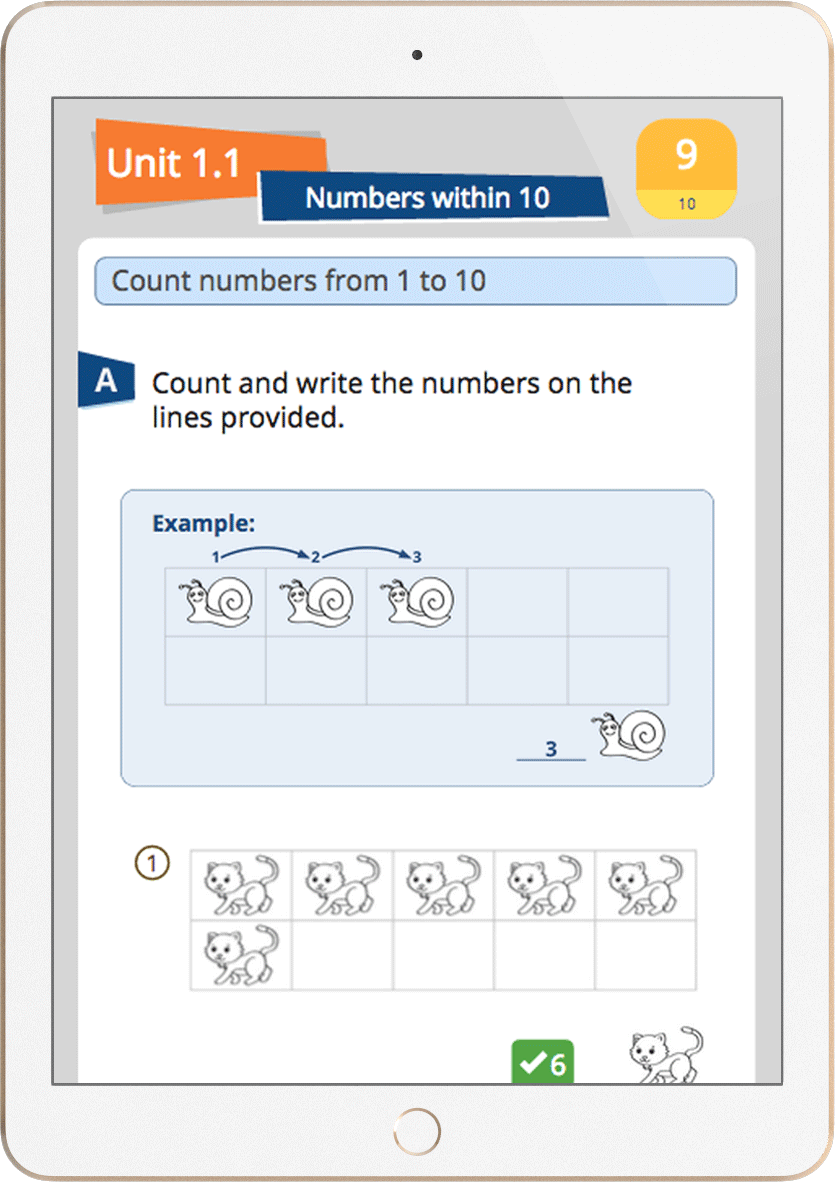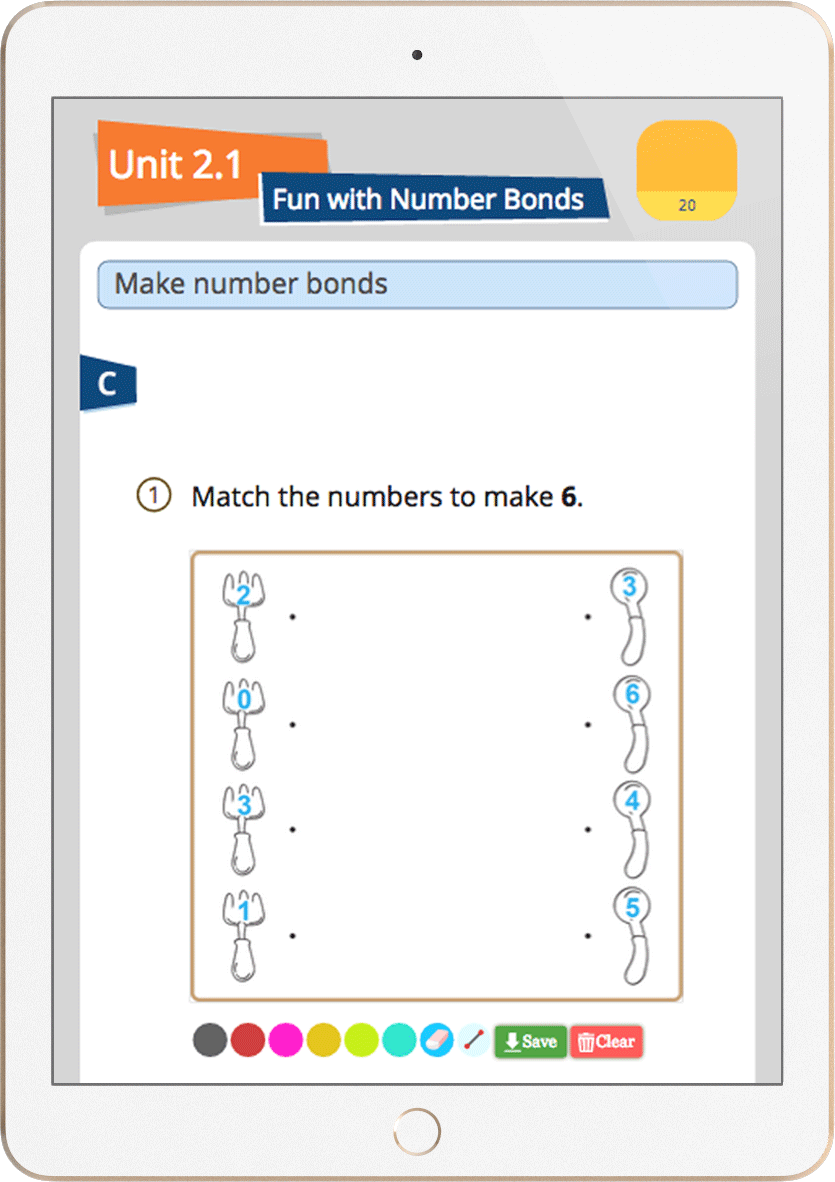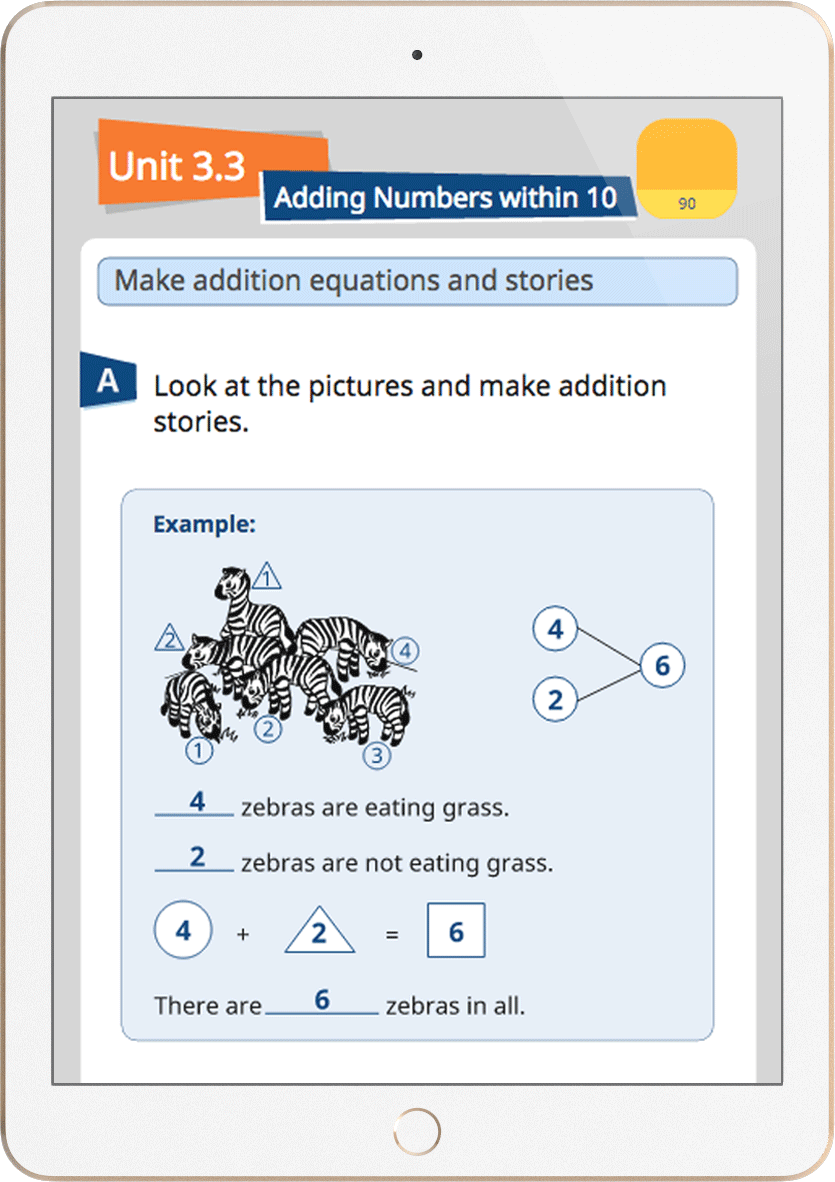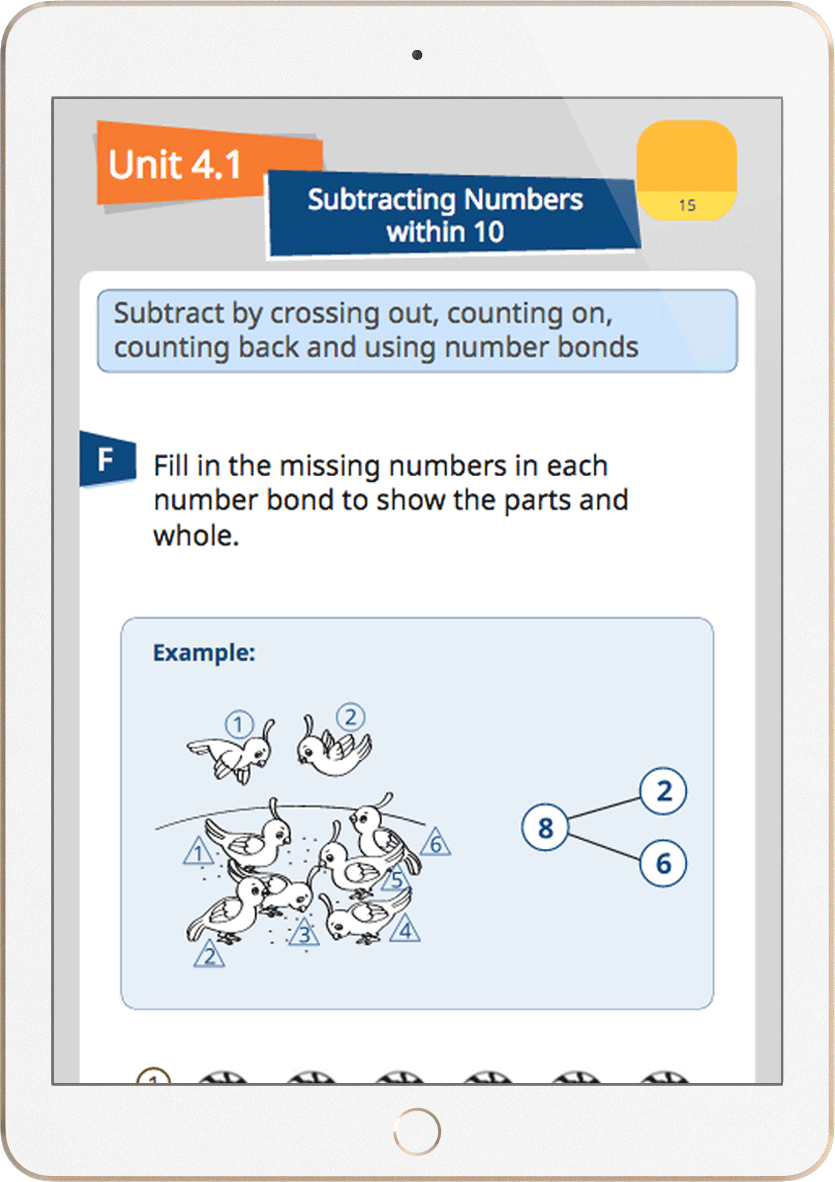LEARNING MATHSSold out

Valid for 2 years

For single user only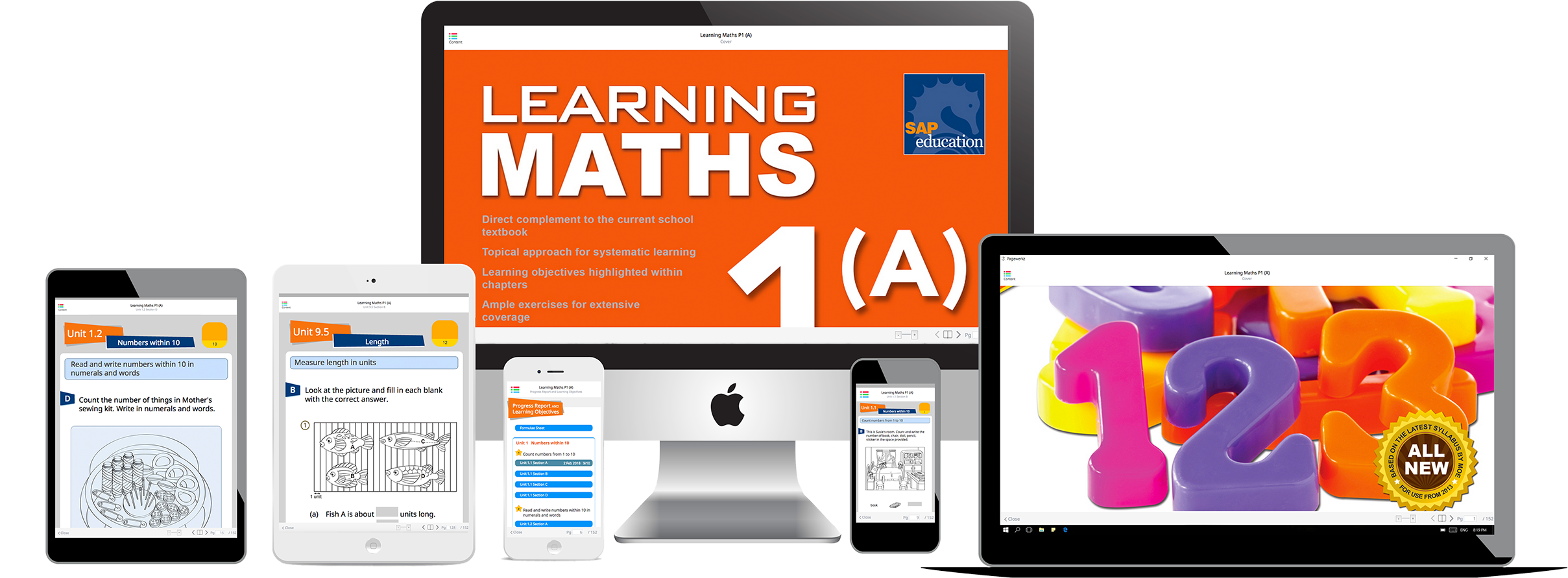Powered by PageWerkz and works on iOS, Android, Windows and Mac with a single purchase.

FREE RESOURCES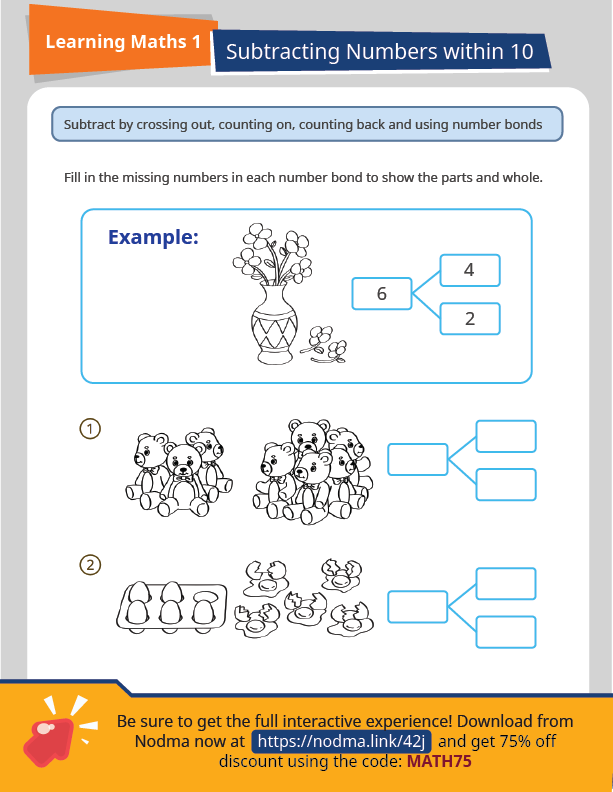Worksheet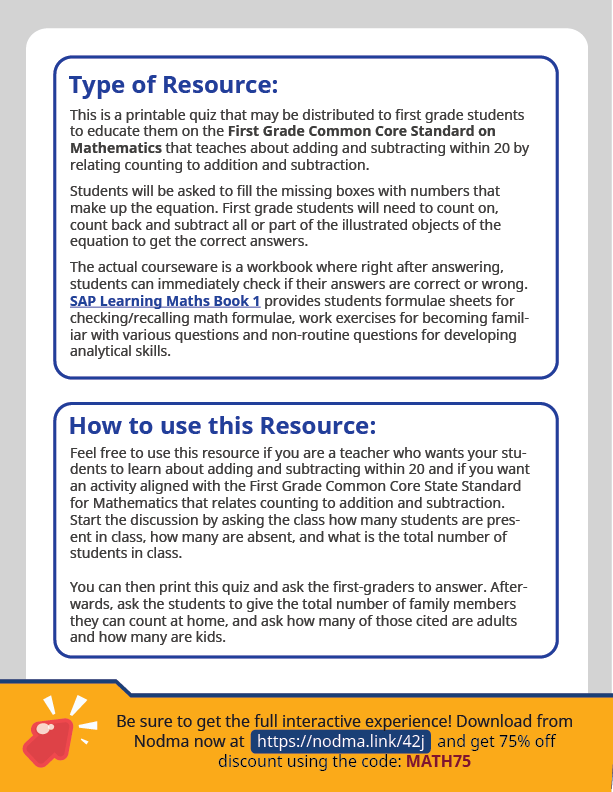Type of Resource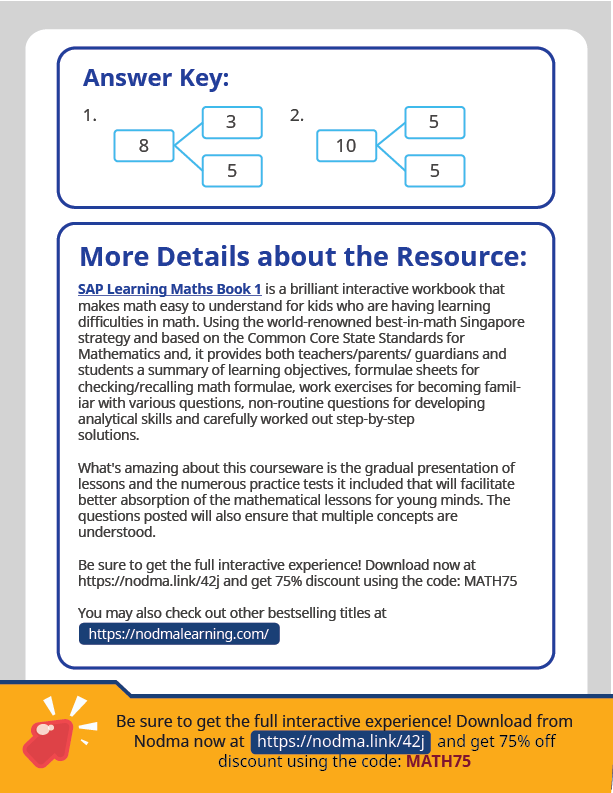Printable Work Sheets

Instructions:

This is a printable quiz that may be distributed to first grade students to educate them on CCSS.MATH.CONTENT.1.OA.C.5, a First Grade Common Core Standard on Mathematics that teaches about adding and subtracting within 20 by relating counting to addition and subtraction.

Students will be asked to fill the missing boxes with numbers that make up the equation. First grade students will need to count on, count back and subtract all or part of the illustrated objects of the equation to get the correct answers.

Printable Work Sheets

Instructions:

This is a printable quiz that may be distributed to first grade students to educate them on CCSS.MATH.CONTENT.1.OA.C.5, a First Grade Common Core Standard on Mathematics that teaches about adding and subtracting within 20 by relating counting to addition and subtraction.

Students will be asked to fill the missing boxes with numbers that make up the equation. First grade students will need to count on, count back and subtract all or part of the illustrated objects of the equation to get the correct answers.WorksheetType of ResourcePrintable Work Sheets

Instructions:

This is a printable quiz that may be distributed to first grade students to educate them on CCSS.MATH.CONTENT.1.OA.C.5, a First Grade Common Core Standard on Mathematics that teaches about adding and subtracting within 20 by relating counting to addition and subtraction.

Students will be asked to fill the missing boxes with numbers that make up the equation. First grade students will need to count on, count back and subtract all or part of the illustrated objects of the equation to get the correct answers.

Printable Work Sheets

Instructions:

This is a printable quiz that may be distributed to first grade students to educate them on CCSS.MATH.CONTENT.1.OA.C.5, a First Grade Common Core Standard on Mathematics that teaches about adding and subtracting within 20 by relating counting to addition and subtraction.

Students will be asked to fill the missing boxes with numbers that make up the equation. First grade students will need to count on, count back and subtract all or part of the illustrated objects of the equation to get the correct answers.